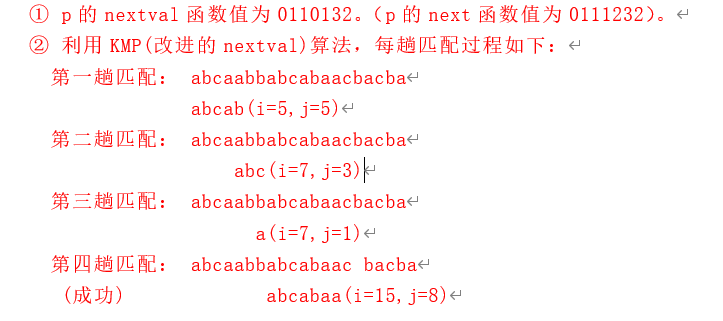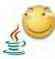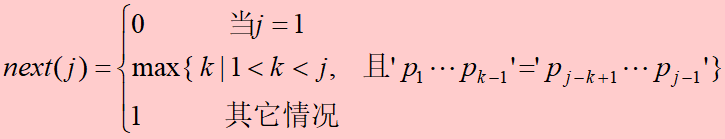### 站点信息### 尼采般地抒情### 站点信息## Brute-Force

①BF算法算是一种暴力算法，首先是查看t的第一字母a和上面s的第一个字母比较相同，所以接着比较比到各自的第三个字符也就是，aba、abc发现不同，

②再递推比较，t回到第一个字母a，这时s回到第二个字符（因为第一个字符已经比过了）相当于babcabcacbab和abcac两个字符串进行比较，很明显第一个字符就不一样，

③再递推比较……

## KMP算法

### 算法详述先学会用，理论日后再补…… 🕊### 计算next函数值

（3）串“ababaaababaa”的next数组为（  ）。

A．012345678999   B．012121111212   C．011234223456    D．0123012322345

 j 1 2 3 4 5 6 7 8 9 10 11 12 t a b a b a a a b a b a a next(j) 0 1 1 2 3 4 2 2 3 4 5 6

①next数组第一位永远是0，1；

②next(j) = 前序列相同元素个数 + 1；

eg：当t = 6：

### 计算next函数修正值

（4）串“ababaabab”的nextval为（  ）。

A．010104101      B．010102101      C．010100011       D．010101011

 j 1 2 3 4 5 6 7 8 9 t a b a b a a b a b next(j) 0 1 1 2 3 4 2 3 4 nextval(j) 0 1 0 1 0 4 1 0 1

①先列举出next(j)，求nextval(j)是基于next(j)的；

③在表格中找出j = x的那一列，如果这一列的t值和②步骤中的t值相同，则结果为j = x这一列的nextval(j)值，如果不相同，则结果为所要求的那一列的next(j)值；

eg：当j = 5时：

eg：当j = 6时：

### 具体匹配情况

（2）设目标为t=“abcaabbabcabaacbacba”,模式为p=“abcabaa”

① 计算模式p的naxtval函数值；

② 不写出算法,只画出利用KMP算法进行模式匹配时每一趟的匹配过程。### 代码

``````#include<bits/stdc++.h>

using namespace std;
typedef int Status;
typedef int ElemType;
#define OVERFLOW -1
#define ERROR 0
#define OK 1

//------串的顺序存储结构-----
#define MAXLEN 225
typedef struct
{
char ch[MAXLEN + 1]; //存储串的一维数组，从下标为 1 的数组分量开始存储的，下标为 0 的分量闲置不用
int length; //串的当前长度
}SString;

//------串的堆式顺序存储结构-----
typedef struct
{
char \*ch; //若是非空串，则按串长分配存储区，否则 ch 为 NULL
int length; //串的当前长度
}HString;

HString S, T;

//-----串的链式存储结构-----
#define CHUNKSIZE 80
typedef struct Chunk
{
char ch[CHUNKSIZE];
struct Chunk *next;
}Chunk;
typedef struct
{
Chunk *head, \*tail; //串的头指针和尾指针
int length; //串的当前长度
}LString;

// //1、生成串
// StrAssign(&T, chars)

// //2、复制
// StrCopy(&T, S)

// //3、判空
// StrEmpty(S)

// //4、比较
// StrCompare(S, T)

// //5、长度
// StrLength(S)

// //6、清空
// ClearString(&S)

// //7、联接
// Concat(&T, S1, S2)

// //8、子串
// SubString(&Sub, S, pos, len)

//9、串的模式匹配\_BF 算法 O(n \* m)
int Index_BF(HString S, HString T, int pos)
{//返回模式 T 在主串 s 中第 pos 个字符开始第一次出现的位置。若不存在，则返回值为 0
//其中，T 非空，1<=pos<=S.length
int i = pos, j = 1; //初始化
while(i <= S.length && j <= T.length) //两串均未比较到串尾
{
if(S.ch[i] == T.ch[j]) //继续比较后继字符
{
i++;
j++;
}
else //指针后退重新开始匹配
{
i = i - j + 2; //i=i-j+1 回到 i 的起点，+2 到下一个字符
j = 1;
}
}
if(j > T.length)
return i - T.length; //匹配成功，返回 T 在 S 中第一次出现的位置
else
return 0;
}

//9、串的模式匹配\_KMP 算法求 next 数组
void get_next(HString, int next[])
{//求模式串 T 的 next 函数值并存入数组 next
int j = 1, t = 0;
next = 0;
while(j < T.length)
{
if(t == 0 || T.ch[j] == T.ch[t])
{
t++;
j++;
next[j] = t;
}
else
t = next[t];
}
}

//9、串的模式匹配\_KMP 算法求 nextval 数组
void get_nextval(HString T, int nextval[])
{//求模式串 T 的 next 函数修正值并存入数组 nextval
int j = 1, t = 0;
nextval = 0;
while(j < T.length)
{
if(t == 0 || T.ch[j] == T.ch[t])
{
t++;
j++;
if(T.ch[j] != T.ch[t])
nextval[j] = t;
else
nextval[j] = nextval[t];
}
else
t = nextval[t];
}
}

//9、串的模式匹配\_KMP 算法 O(n + m)
int Index_KMP(HString S, HString T, int pos, int next[])
{//利用模式串 T 的 next 函数求 T 在主串 S 中第 pos 个字符之后的位置
//其中，T 非空，1<=pos<=S.length
int i = pos, j = 1;
while(i <= S.length && j <= S.length) //两个串均未比较到串尾
{
if(j == 0 || S.ch[i] == T.ch[i]) //继续比较后继字符
{
i++;
j++;
}
else
j = next[j]; //模式串向右移动
if(j > T.length) //匹配成功
return i - T.length;
else
return 0;
}
}

// //10、插入
// Strlnsert(&S, pos, T)

// //11、删除
// StrDelete(&S, pos, len)

// //12、销毁
// DestroyString(&S)

``````

Twikoo giscus